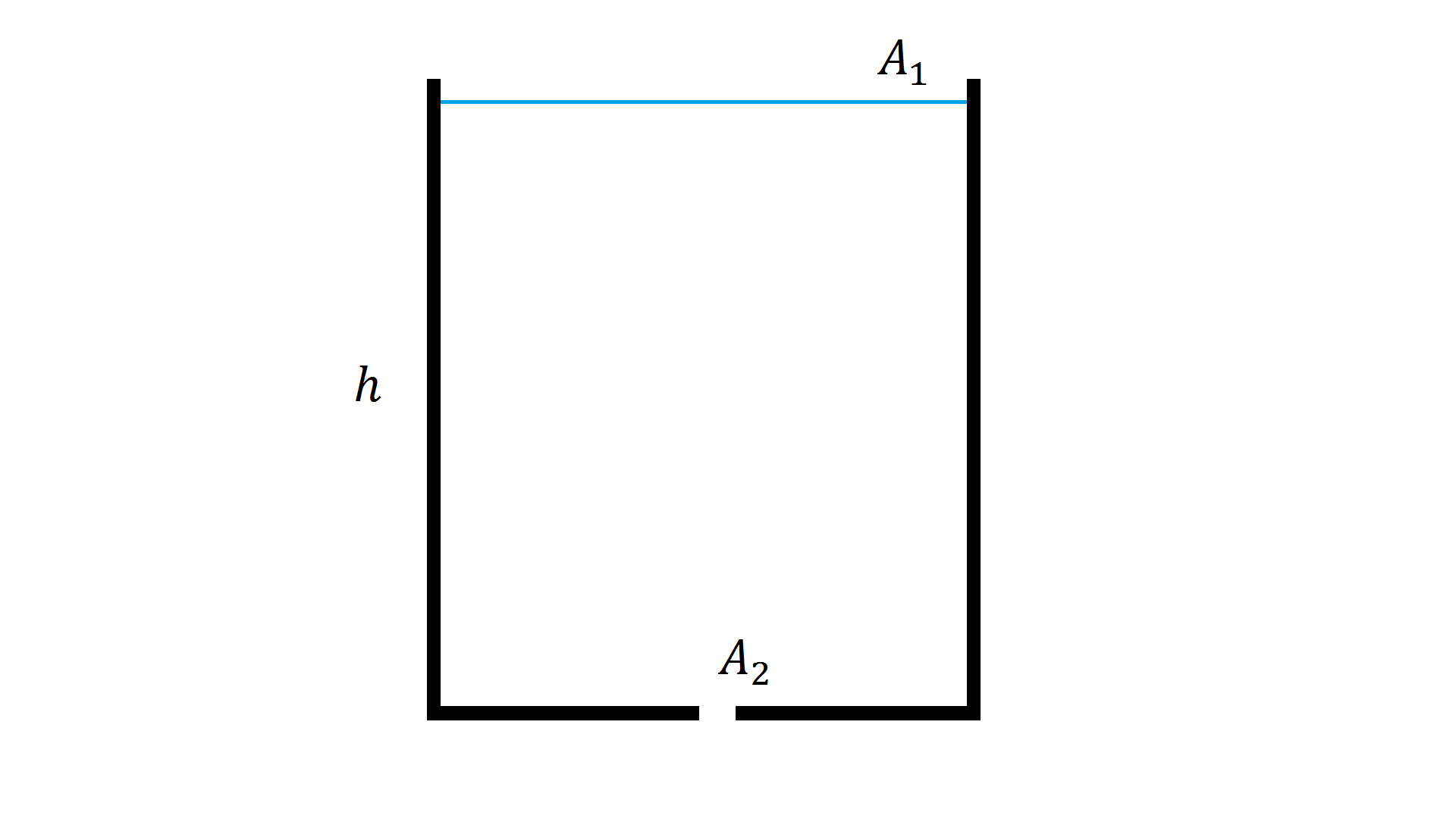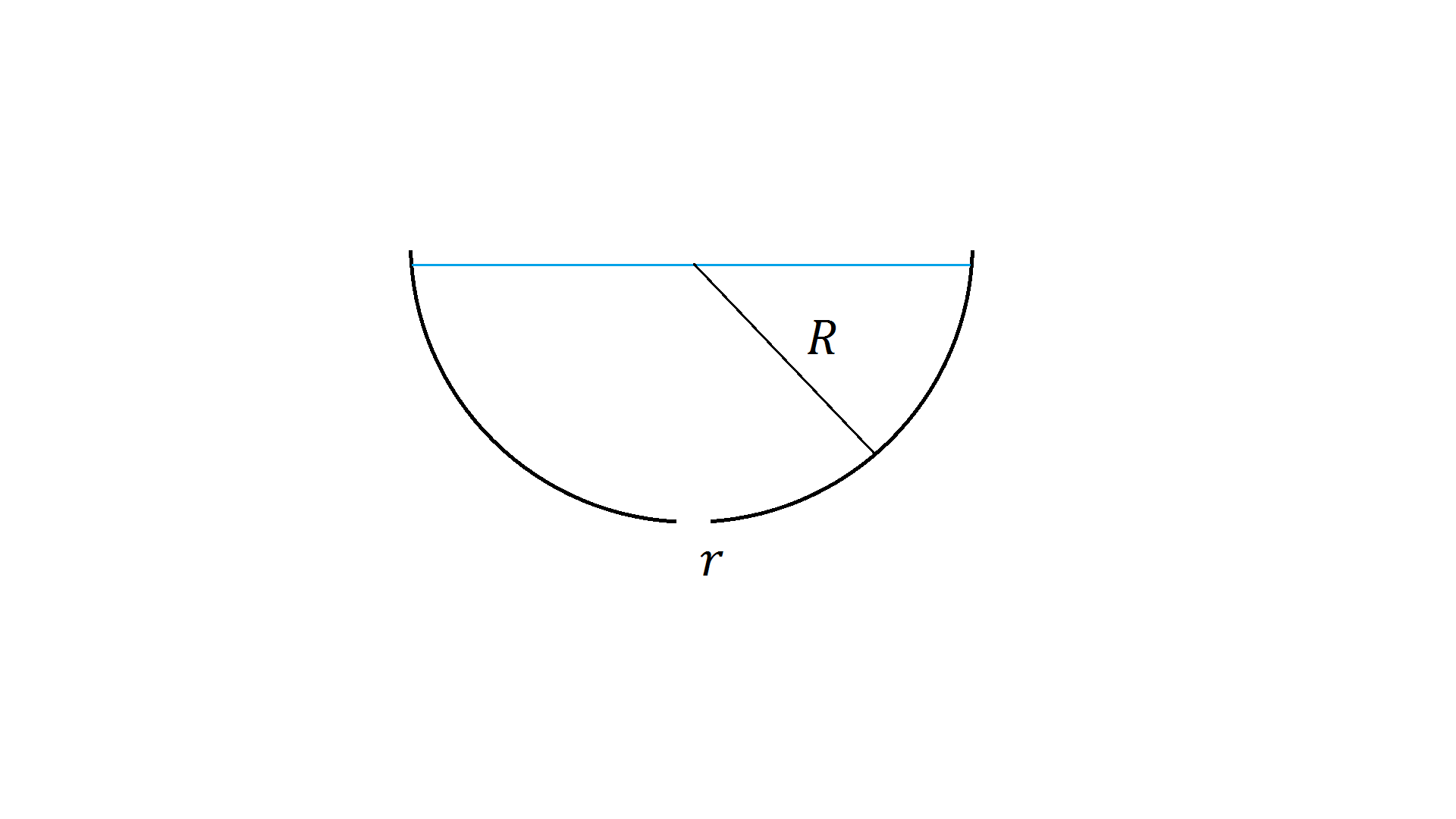# Draining a Container

Back

Draining a Container

Question: Consider a container with area$A_1$ and height$h$. A small hole of$A_2$ is put into the bottom of the container. How much time$t$ does it take to drain the container? Assume$A_2 \ll A_1$.We will show:$t = \frac{A_1}{A_2} \sqrt{\frac{2h}{g}}$

Applicable Ideas:

Continuity Equation$A_1 v_1 = A_2 v_2$

There is no accumulation in the amount of fluid anywhere in the container.

Bernoulli Equation$P_1 + \frac{1}{2} \rho {v_1}^2 + \rho g h_1 = P_2 + \frac{1}{2} \rho {v_2}^2 + \rho g h_2$

The statement of conservation of energy for the fluid. Applying this to the problem, where$P_o$ is atmospheric pressure:$P_o + \frac{1}{2} \rho {v_1}^2 + \rho g h = P_o + \frac{1}{2} \rho {v_2}^2$$\frac{1}{2} \rho {v_1}^2 + \rho g h = \frac{1}{2} \rho {v_2}^2$${v_1}^2 + 2gh = {v_2}^2$$v_1 = \frac{dh}{dt}$

Sidenote: If$A_1 \gg A_2$, then$v_1 \ll v_2$ and we have$v_2 = \sqrt{2gh}$. This is Torricelli’s law where the speed of the fluid emerging from the bottom of the container is equal to the speed it would have attained falling through a distance$h$.

Here we can use that fact to write:$A_1 \frac{dh}{dt} = - A_2 \sqrt{2gh}$

The negative sign is introduced because the water is leaving the container.$\frac{dh}{dt} = - \frac{A_2}{A_1} \sqrt{2gh}$$\int^0_h \frac{1}{\sqrt{h}}\,dh = \sqrt{2g} \frac{A_2}{A_1} \int^t_0\,dt$$2\sqrt{h} = \sqrt{2g} \frac{A_2}{A_1} t$$t = \frac{A_1}{A_2} \sqrt{\frac{2h}{g}}$

Bonus Question: Consider a hemispherical container of radius$R$. A small hole of radius$r = 1$ is put into the bottom of the container. How much time$t$ does it take to drain the container? Assume$r \ll R$.We will show:$t = \frac{14}{15}\frac{R^{5/2}}{r^2\sqrt{2g}}$$r = 1$$t = \frac{14}{15}\frac{R^{5/2}}{\sqrt{2g}}$

We will apply the continuity equation and Bernoulli’s equation just like before.$R(t)^2 = R^2 - (R - h)^2 = 2Rh - h^2$$A_1 = \pi R(t)^2 = \pi (2Rh - h^2)$$A_2 = \pi r^2$

From the Bernoulli equation:${v_1}^2 + 2gR = {v_2}^2$

We will approximate${v_2}^2 = 2gR$ because$A_1 \gg A_2$ holds most of the time.$\pi (2Rh - h^2) \frac{dh}{dt} = - \pi r^2 \sqrt{2gh}$$\frac{h^2 - 2Rh}{\sqrt{h}} dh = r^2 \sqrt{2g}\,dt$$\frac{1}{r^2\sqrt{2g}}\int^R_0 2Rh^{1/2} - h^{3/2}\,dh = \int^t_0 \,dt$$\left[\frac{4}{3}Rh^{3/2} - \frac{2}{5}h^{5/2}\right]^R_0 = \frac{4}{3}R^{5/2} - \frac{2}{5}R^{5/2}$$\frac{14}{15}R^{5/2} = t r^2 \sqrt{2g}$$t = \frac{14}{15}\frac{R^{5/2}}{r^2\sqrt{2g}}$$r = 1$$t = \frac{14}{15}\frac{R^{5/2}}{\sqrt{2g}}$